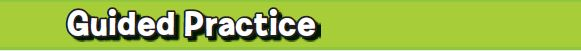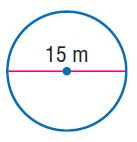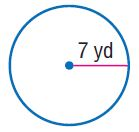Homework Explained - Math Practice 101Dear guest, you are not a registered member. As a guest, you only have read-only access to our books, tests and other practice materials.

As a registered member you can:

Registration is free and doesn't require any type of payment information. Click here to Register.
Go to page:
Chapter 8: Measure Figures; Lesson 1: Circumference

###Find the radius or diameter of each circle with the given dimension.

•  $$r=$$ $$\text{m}$$
•  $$d=$$ $$\text{ft}$$
•  $$r=$$ $$\text{in}$$

Find the circumference of each circle. Use 3.14 or$$\frac{22}{7}$$ for $$\pi$$. Round to the nearest tenth if necessary.

• Question 4•  $$C\approx$$ $$\text{m}$$
• Question 5•  $$C\approx$$ $$\text{yd}$$
• Question 6

Building on the Essential Question A circle has a circumference of about 16.3 meters and a diameter of about 5.2 meters. What is the relationship between the circumference and diameter of this circle?

• Type below:

Yes, email page to my online tutor. (if you didn't add a tutor yet, you can add one here)